#### Vol. 18, No. 1, 1966

 Download this articleFor screen For printingRecent Issues Vol. 311: 1 Vol. 310: 1  2 Vol. 309: 1  2 Vol. 308: 1  2 Vol. 307: 1  2 Vol. 306: 1  2 Vol. 305: 1  2 Vol. 304: 1  2Online Archive Volume: Issue:The Journal Subscriptions Editorial Board Officers Contacts Submission Guidelines Submission Form Policies for Authors ISSN: 1945-5844 (e-only) ISSN: 0030-8730 (print) Special Issues Author Index To Appear Other MSP Journals
On the characteristic roots of the product of certain rational integral matrices of order two

### Lorraine L. Foster

Vol. 18 (1966), No. 1, 97–110
##### Abstract

This paper deals with a special case of the following problem: Let A, B be matrices of order n over the rational integers. Compare the algebraic number field generated by the characteristic roots of AB with those generated by A, B.

We let M(r,s) denote the companion matrix of x2 + rx + s, for rational integers r and s, and let N(r,s) = M(r,s)(M(r,s)). Further let F(M(r,s)) and F(N(r,s)) denote the fields generated by the characteristic roots of M(r,s) and N(r,s) over the rational field, R. This paper is concerned with F(N(r,s)), especially in relation to F(M(r,s)). The principal results obtained are outlined as follows:

Let S be the set of square-free integers which are sums of two squares. Then F(N(r,s)) is of the form R(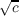) where c S. Further, F(N(r,s)) = R if and only if rs = 0. Suppose c S. Then there exist infinitely many distinct pairs of integers (r,s) such that F(N(r,s)) = R(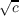).

Further, if c S, there exists an infinite sequence {(rn,sn)} of distinct pairs of integers such that F(M(rn,sn)) = R(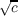) and F(N(r n,sn)) = R(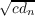) for some integers dn such that (c,dn) = 1. If c S and c is odd or c = 2, there exists an infinite sequence {(rn,sn)} of distinct pairs of integers such that F(N(rn,sn)) = R(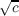) and F(M(r n,sn)) = R(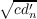) for some integers dnsuch that (c,dn) = 1.

There are five known pairs of integers (r,s) with rs0 and s 1 such that F(M(r,s)) and F(N(r,s)) coincide. For s 2 (mod 4) and for certain odd integers s the fields F(M(r,s)) and F(N(r,s)) cannot coincide for any integers r.

Finally, for any integer r0 (or s0,1) there exist at most a finite number of integers s (or r) such that the two fields coincide.

Primary: 10.79
Secondary: 15.48
##### Milestones